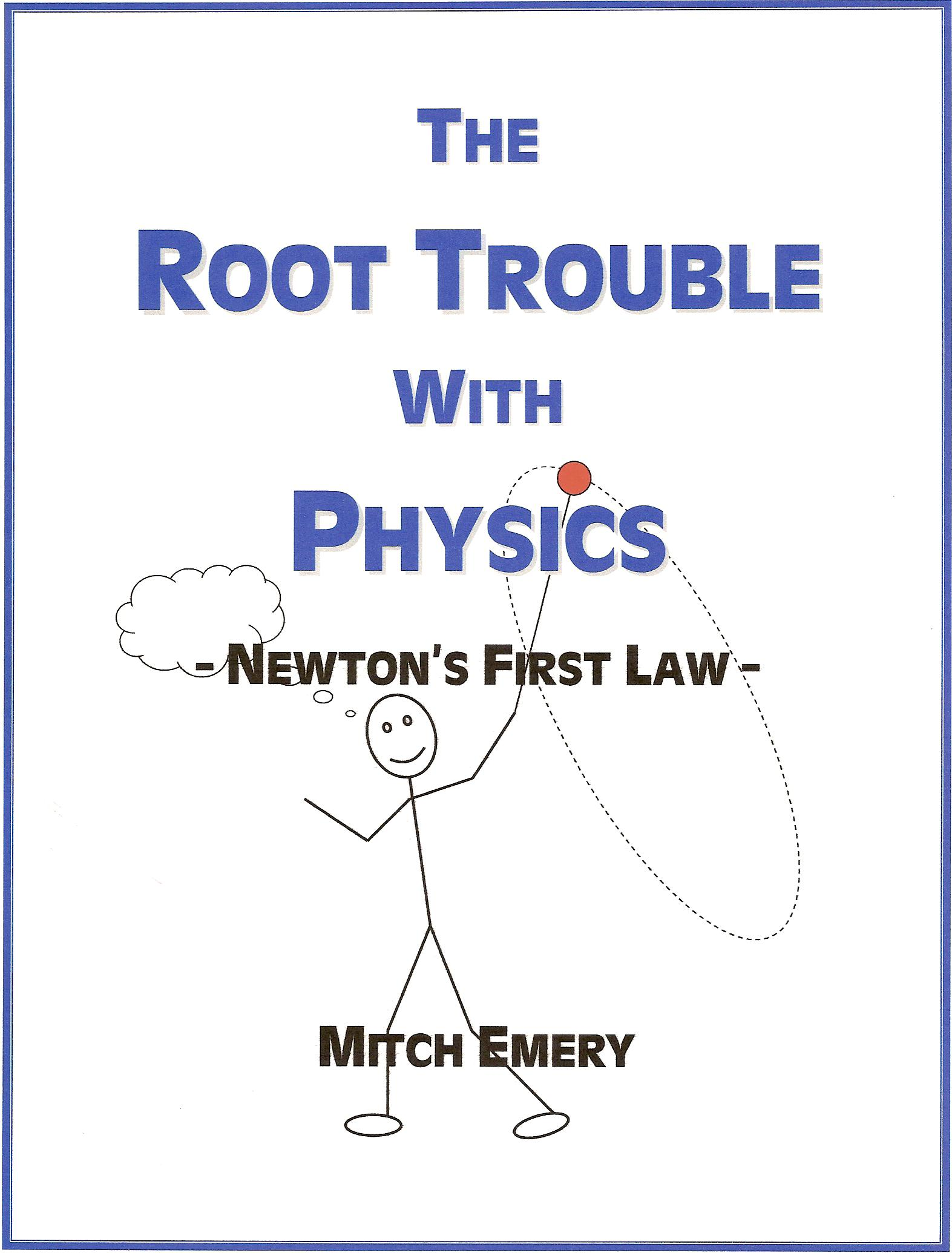Enter the content which will be displayed in sticky bar

# BookPages: 26
Year: 2008

The Root Trouble with Physics: Newtons First Law

This booklet has all the ingredients for a scientific revolution. Newton\'s first law states that a body in motion tends to move in a straight line at a constant speed unless acted upon by an external force. Indeed the first law seems valid, but is it really so? In his second law, Newton said if a body is acted upon by an unbalanced force, then there will be a change in its velocity proportional to the magnitude of the force and in the same direction in which the force is applied. But there is more to the story. Newton\'s laws of motion describe relationships between external forces acting on a body and the motion of the body, but they do not consider effects of force from within a body. When a body is pulled into a circular path of travel, the pulling force creates tension throughout the body. Due to this tension, the body\'s momentum is held at a right angle to the force. Subsequently, the body works to break away from the force so as to move in a straight line, but the pull away has nothing to do with Newton\'s first law. The force causes it. Yet as formulated by Newton, the first law is more than a special case of the second law. The importance of the first law is to establish frames of reference for which the other laws are applicable, such frames being called \'inertial\' frames. But to say the first law is verified by the second law is simply not true. Motion in a straight line could be a special case of the second law, while generally everything moves about inertial frames of natural and force-free circular motion.

These basic principles of motion lead to reinterpretations of relativity theory, quantum mechanics, big bang theory, and cosmology. In consideration of Occam\'s razor - what could be simpler? A proper understanding of inertia brings about a radical change in physics, and then goes on to explain the Universe in a better way.